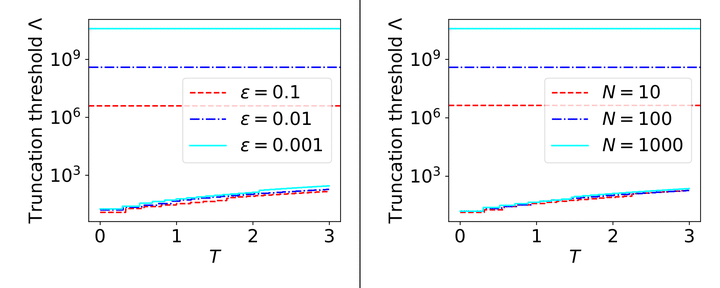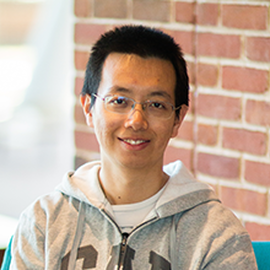# Provably accurate simulation of gauge theories and bosonic systemsImage credit: Yu Tong

### Abstract

Quantum many-body systems involving bosonic modes or gauge fields have infinite-dimensional local Hilbert spaces which must be truncated to perform simulations of real-time dynamics on classical or quantum computers. To analyze errors resulting from truncation, we develop methods for bounding the rate of growth of local quantum numbers such as the occupation number of a mode at a lattice site, or the electric field at a lattice link. Our approach applies to various models of bosons interacting with spins or fermions such as the Hubbard-Holstein, Fröhlich, and Dicke models, and also to both abelian and non-abelian gauge theories. We show that if states in these models are truncated by imposing an upper limit $\Lambda$ on each local quantum number, and if the initial state has low local quantum numbers, then a truncation error no worse than $\epsilon$ can be achieved by choosing $\Lambda$ to increase polylogarithmically with $\epsilon^{-1}$, an exponential improvement over previous bounds based on energy conservation. For the Hubbard-Holstein model, we numerically compute an upper bound on the value of $\Lambda$ that achieves accuracy $\epsilon$, finding significant improvement over previous estimates in various parameter regimes. We also establish a criterion for truncating the Hamiltonian with a provable guarantee on the accuracy of time evolution. Building on that result, we formulate quantum algorithms for dynamical simulation of lattice gauge theories and of models with bosonic modes; the gate complexity depends almost linearly on spacetime volume in the former case, and almost quadratically on time in the latter case. We establish a lower bound showing that there are systems involving bosons for which this quadratic scaling with time cannot be improved. By applying our results on the truncation error in time evolution, we also prove that spectrally isolated energy eigenstates can be approximated with error at most $\epsilon$ by truncating local quantum numbers at $\Lambda=\textrm{polylog}(\epsilon^{-1})$.

Type
Publication
Quantum 6, 816 (2022)##### Yuan Su
###### Senior Researcher

I work on quantum algorithms for simulating Hamiltonian dynamics. I am particularly interested in the design, analysis, implementation, and application of quantum simulation.